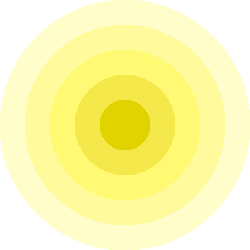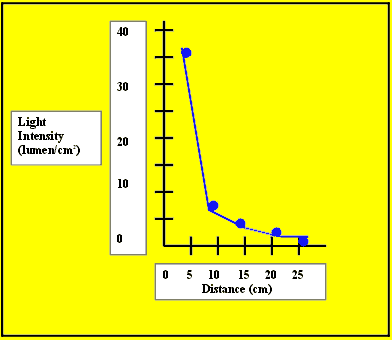Measure How a Light Beam Diminishes with Distance

You probably know that light from a headlight or a flashlight diminishes with distance. For example, at night your headlights only let you see a certain distance down the road. Beyond that distance, everything is black. In this experiment you will learn how much the light diminishes and why!

What you need for this experiment

You will need:

• A flat table
• A small flashlight with a beam you can focus
• Some books to raise the flashlight off the tabletop
• A ruler to measure distance
• A pencil
• Another book with a sheet of white paper taped to the front

Performing the experiment

We are going to trace the circle of light created by shining the flashlight at the paper from increasing distances.

To create the circles:

• Set the flashlight on a stack of books, turn it on and focus the beam as small as you can.
• Using the ruler, measure distances of 5, 10, 15, 20 and 25 centimeters from the front of the flashlight and mark these distances on the tabletop with pieces of tape.
• Place the white paper taped to the book at the 5 cm mark and trace the circle of the focused beam.
• Move the white paper to the 10 cm mark and see how the beam circle became bigger! Trace the circle of the focused beam; do not worry if it is not a perfect circle.
• Continue to move the paper to each mark and trace the circle of the beam.As the circles become bigger, the light becomes dimmer.

To analyze your data:

• Make a data table with the columns of distance (cm), diameter (cm), area (cm2) and intensity (lumen/cm2).
• Fill in the distance column with your numbers (i.e., 5-25).
• Measure the diameter of each circle and fill in the diameter column with the measurements for the corresponding distances.
• Calculate the area of each circle by using the formula for the area of a circle in terms of the diameter (area = ¼*pi*diameter2 = 0.785*diameter2) and fill in the area column for each corresponding distance.
• Since we do not know the exact output of the flashlight, let's just assume a convenient number (100 lumens). For the purposes of this example, the value really does not matter because the output is constant. Calculate the intensity for each distance by dividing 100 lumens by the area of the circle.

We performed this experiment and obtained the following results:

 Distance (cm) Diameter (cm) Area (cm2) Intensity (lumen/cm2) 10 4.1 13.2 7.6 15 6.3 31.2 3.2 20 8.0 50.2 2.0 25 9.6 72.4 1.3

If you wish, you can make a graph with the distance on the horizontal axis and the intensity on the vertical axis. When we graphed the change in light intensity with distance, look at what we found.What actually happened

When you moved the paper away from the flashlight, the circle of the beam (i.e., the area) became much larger. Because the light output of the beam was constant, the intensity of the beam became much less. In fact, you can see in the graph that, as you doubled the distance from the flashlight, the intensity decreased by 4 times (i.e., 2 squared). So, to collect all of the light from the beam, you would need a bigger circle or "eye."